## How to Calculate and Solve for Net Heat Flux for Radiation | Radiation Heat Transfer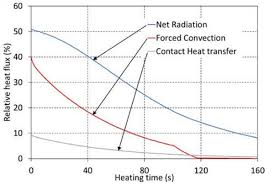The image above represents net heat flux for radiation.

To compute for net heat flux for radiation, four essential parameters are needed and these parameters are Emissivity (ε), Area (A), Emitting Power of Black Body (eb) and Radiosity (J).

The formula for calculating net heat flux for radiation:

qnet = εAeb / 1 – ε – J

Where:

qnet = Net Heat Flux for Radiation
ε = Emissivity
A = Area
eb = Emitting Power of Black Body

Let’s solve an example;
Find the net heat flux for radiation when the emissivity is 22, the area is 2, the emitting power of black body is 10 and the radiosity is 14.

This implies that;

ε = Emissivity = 22
A = Area = 2
eb = Emitting Power of Black Body = 10

qnet = εAeb / 1 – ε – J
qnet = (22)(2)(10) / 1 – 22 – 14
qnet = 440 / -21 – 14
qnet = -20.9523 – 14
qnet = -34.95

Therefore, the net heat flux for radiation is -34.95 W.

## How to Calculate and Solve for Emissive Power | Radiation Heat Transfer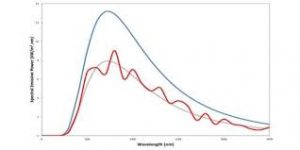The image above represents emissive power.

To compute for emissive power, two essential parameters are needed and these parameters are Area of Emitting Body (A) and Geometric Body Constant (F).

The formula for calculating emissive power:

Q = AF

Where:

Q = Emissive Power
A = Area of Emitting Body
F = Geometric Body Constant

Let’s solve an example;
Find the emissive power when the area of emitting body is 12 and the geometric body constant is 6.

This implies that;

A = Area of Emitting Body = 12
F = Geometric Body Constant = 6

Q = AF
Q = 12(6)
Q = 72

Therefore, the emissive power is 72 W.

Calculating the Area of Emitting Body when the Emissive Power and the Geometric Body Constant is Given.

A = Q / F

Where:

A = Area of Emitting Body
Q = Emissive Power
F = Geometric Body Constant

Let’s solve an example;
Find the area of emitting body when the emissive power is 32 and the geometric body constant is 8.

This implies that;

Q = Emissive Power = 32
F = Geometric Body Constant = 8

A = Q / F
A = 32 / 8
A = 4

Therefore, the area of emitting body is 4.

## How to Calculate and Solve for Total Irradiation | Radiation Heat Transfer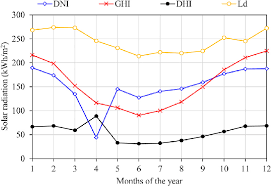The image above represents total irradiation.

To compute for total irradiation, three essential parameters are needed and these parameters are Reflectivity (ρ), Emissive Power of First Body (e) and Emissive Power of Second Body (e2).

The formula for calculating total irradiation:

G = e2 + ρe / 1 – ρ

Where:

ρ = Reflectivity
e = Emissive Power of First Body
e2 = Emissive Power of Second Body

Let’s solve an example;
Given that the reflectivity is 21, the emissive power of first body is 3 and the emissive power of second body is 9. Find the total irradiation?

This implies that;

ρ = Reflectivity = 21
e = Emissive Power of First Body = 3
e2 = Emissive Power of Second Body = 9

G = e2 + ρe / 1 – ρ
G = 9 + 21(3) / 1 – 21
G = 9 + 63 / -20
G = 72 / -20
G = -3.6

Therefore, the total irradiation is -3.6.

## How to Calculate and Solve for Stefan Boltzmann’s Constant | Radiation Heat Transfer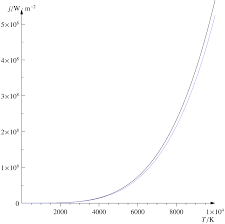The image above represents stefan boltzmann’s constant.

To compute for stefan boltzmann’s constant, three essential parameters are needed and these parameters are Boltzmann’s Constant (KB), Planck’s Constant (h) and Speed of Light (c).

The formula for calculating stefan boltzmann’s constant:

σ = 2π5KB4 / 15c²h5

Where:

σ = Stefan Boltzmann’s Constant
KB = Boltzmann’s Constant
h = Planck’s Constant
c = Speed of Light

Let’s solve an example;
Find the stefan boltzmann’s constant when the boltzmann’s constant is 42, the planck’s constant is 34 and the speed of light is 26.

This implies that;

KB = Boltzmann’s Constant = 42
h = Planck’s Constant = 34
c = Speed of Light = 26

σ = 2π5KB4 / 15c²h5
σ = 2π5(42)4 / 15(26)²(34)5
σ = 2(306.019)(3111696) / 15(676)(45435424)
σ = 1904480458.135 / 460715199360
σ = 0.00413

Therefore, the stefan boltzmann’s constant is 0.00413.

## How to Calculate and Solve for Monochromatic Emissive Power | Radiation Heat Transfer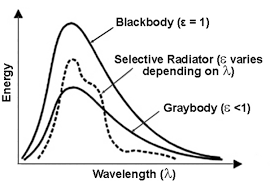The image above represents monochromatic emissive power.

To compute for monochromatic emissive power, five essential parameters are needed and these parameters are Planck’s Constant (h), Velocity of Light (c), Wavelength (λ), Boltzmann’s Constant (KB) and Temperature (T).

The formula for calculating monochromatic emissive power:

ebx = 2πhc²λ-5 / exp(ch/KBλT) – 1

Where:

ebx = Monochromatic Emissive Power | Planck’s Equation
h = Planck’s Constant
c = Velocity of Light
λ = Wavelength
KB = Boltzmann’s Constant
T = Temperature

Let’s solve an example;
Find the monochromatic emissive power when the planck’s constant is 6.626E-24, the velocity of light is 3E8, the wavelength is 22, the boltzmann’s constant is 1.380E-23 and the temperature is 10.

This implies that;

h = Planck’s Constant = 6.626E-24
c = Velocity of Light = 3E8
λ = Wavelength = 22
KB = Boltzmann’s Constant = 1.380E-23
T = Temperature = 10

ebx = 2πhc²λ-5 / exp(ch/KBλT) – 1
ebx = 2π(6.62607004e-34)(300000000)²(22)-5 / exp((300000000)(6.62607004e-34)/(1.38064852e-23)(22)(10)) – 1
ebx = 2π(6.62607004e-34)(90000000000000000)(1.94037e-7) / exp(1.987821012e-25/3.037426744e-21) – 1
ebx = 7.270512005456302e-23 / exp(0.00006544424539379114) – 1
ebx = 7.270512005456302e-23 / 1.0000654463869152 – 1
ebx = 7.270512005456302e-23 / 0.00006544638691519111
ebx = 1.11e-18

Therefore, the monochromatic emissive power is 1.11e-18.

## How to Calculate and Solve for Energy Transfer by Radiation | Radiation Heat Transfer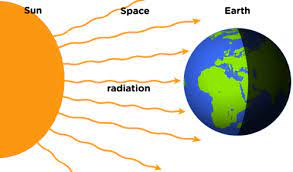The image above represents energy transfer by radiation.

To compute for energy transfer by radiation, three essential parameters are needed and these parameters are Emissivity (ε1), Absorbivity (α1) and Emissive Power of Black Body (eb).

The formula for calculating energy transfer by radiation:

qnet = eb1 – α1)

Where:

qnet = Energy Transfer by Radiation
ε1 = Emissivity
α1 = Absorbivity
eb = Emissive Power of Black Body

Let’s solve an example;
Find the energy transfer by radiation when the emissivity is 30, the absorbivity is 10 and the emissive power of black body is 4.

This implies that;

ε1 = Emissivity = 30
α1 = Absorbivity = 10
eb = Emissive Power of Black Body = 4

qnet = eb1 – α1)
qnet = 4(30 – 10)
qnet = 4(20)
qnet = 80

Therefore, the energy transfer by radiation is 80 J.

Calculating the Emissivity when the Energy Transfer by Radiation, the Aborbivity and the Emissive Power of Black Body is Given.

ε1 = (qnet / eb) + α1

Where:

ε1 = Emissivity
qnet = Energy Transfer by Radiation
α1 = Absorbivity
eb = Emissive Power of Black Body

Let’s solve an example;
Find the emissivity when the energy transfer by radiation is 28, the absorbivity is 3 and the emissive power of black body is 7.

This implies that;

qnet = Energy Transfer by Radiation = 28
α1 = Absorbivity = 3
eb = Emissive Power of Black Body = 7

ε1 = (qnet / eb) + α1
ε1 = (28 / 7) + 3
ε1 = 4 + 3
ε1 = 7

Therefore, the emissivity is 7

Calculating the Aborbivity when the Energy Transfer by Radiation, the Emissivity and Emissive Power of Black Body is Given.

α1 = ε1 – (qnet / eb)

Where:

α1 = Absorbivity
qnet = Energy Transfer by Radiation
ε1 = Emissivity
eb = Emissive Power of Black Body

Let’s solve an example;
Find the absorbivity when the energy transfer by radiation is 8, the emissivity is 18 and the emissive power of black body is 4.

This implies that;

qnet = Energy Transfer by Radiation = 8
ε1 = Emissivity = 18
eb = Emissive Power of Black Body = 4

α1 = ε1 – (qnet / eb)
α1 = 18 – (8 / 4)
α1 = 18 – 2
α1 = 16

Therefore, the absorbivity is 16.

Calculating the Emissive Power of Black Body when the Energy Transfer by Radiation, the Emissivity and Absorbivity is Given.

eb = qnet / ε1 – α1

Where:

eb = Emissive Power of Black Body
qnet = Energy Transfer by Radiation
ε1 = Emissivity
α1 = Absorbivity

Let’s solve an example;
Find the emissive power of black body when the energy transfer by radiation is 50, the emissivity is 15 and the absorbivity is 10.

This implies that;

qnet = Energy Transfer by Radiation = 50
ε1 = Emissivity = 15
α1 = Absorbivity = 10

eb = qnet / ε1 – α1
eb = 50 / 15 – 10
eb = 50 / 5
eb = 10

Therefore, the emissive power of black body is 10.

Nickzom Calculator – The Calculator Encyclopedia is capable of calculating the energy transfer by radiation.

To get the answer and workings of the energy transfer by radiation using the Nickzom Calculator – The Calculator Encyclopedia. First, you need to obtain the app.

You can get this app via any of these means:

To get access to the professional version via web, you need to register and subscribe for NGN 2,000 per annum to have utter access to all functionalities.
You can also try the demo version via https://www.nickzom.org/calculator

Apple (Paid) – https://itunes.apple.com/us/app/nickzom-calculator/id1331162702?mt=8
Once, you have obtained the calculator encyclopedia app, proceed to the Calculator Map, then click on Materials and Metallurgical under Engineering.Now, Click on Radiation Heat Transfer under Materials and Metallurgical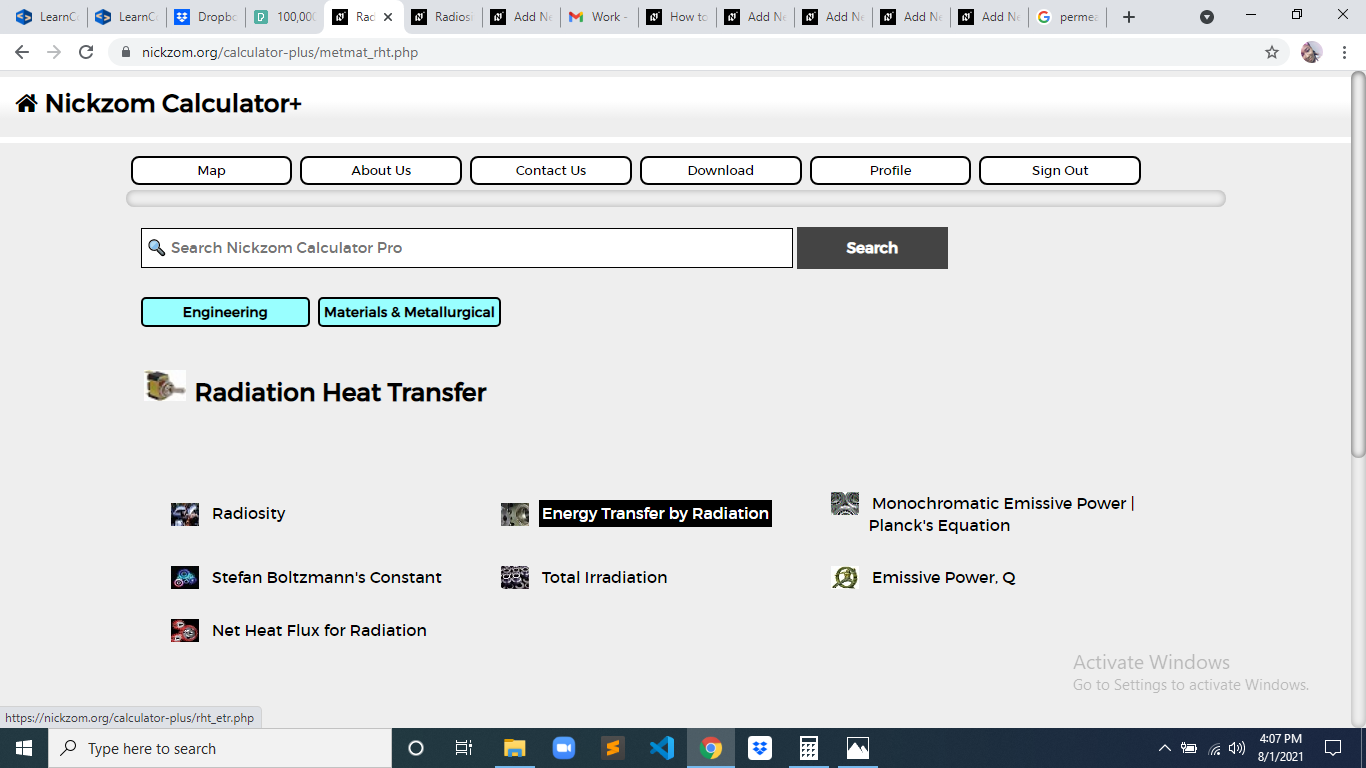The screenshot below displays the page or activity to enter your values, to get the answer for the energy transfer by radiation according to the respective parameter which is the Emissivity (ε1), Absorbivity (α1) and Emissive Power of Black Body (eb).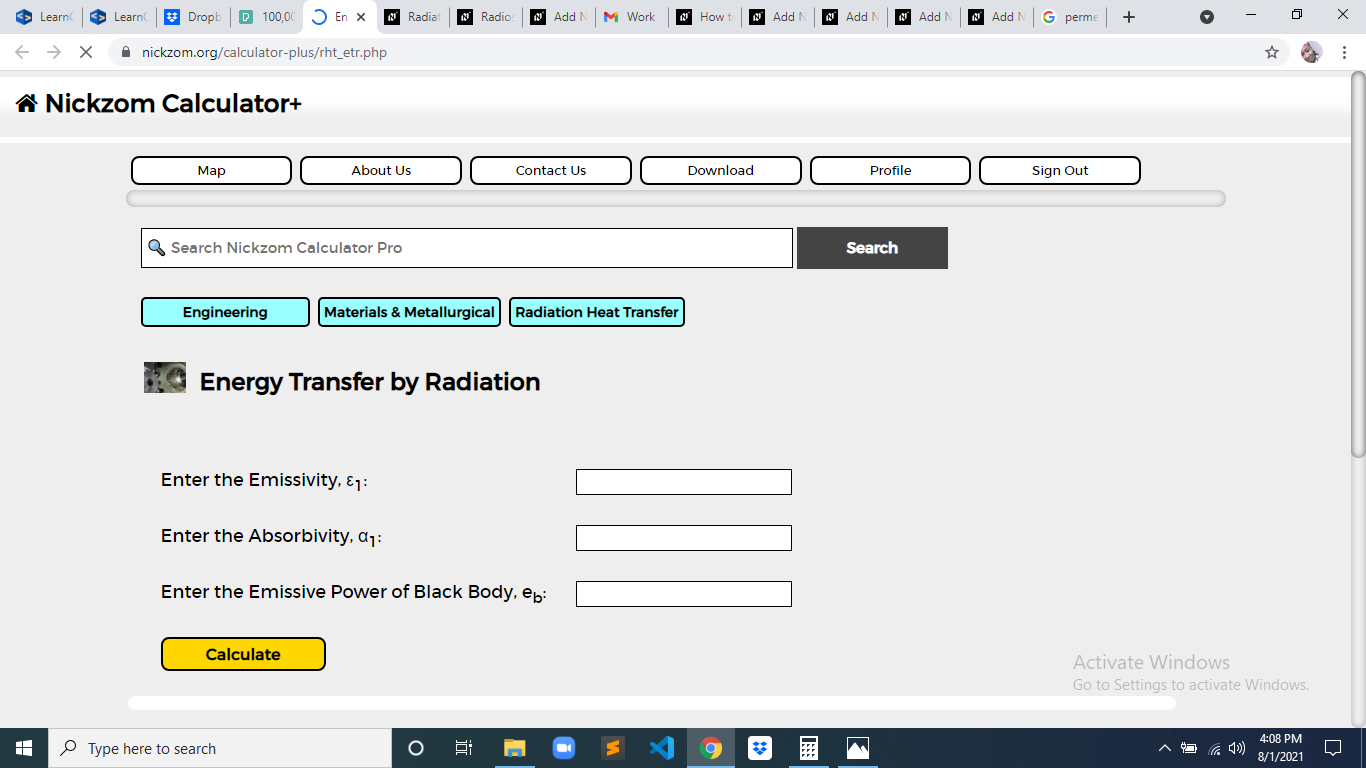Now, enter the values appropriately and accordingly for the parameters as required by the Emissivity (ε1) is 30, Absorbivity (α1is 10 and Emissive Power of Black Body (eb) is 4.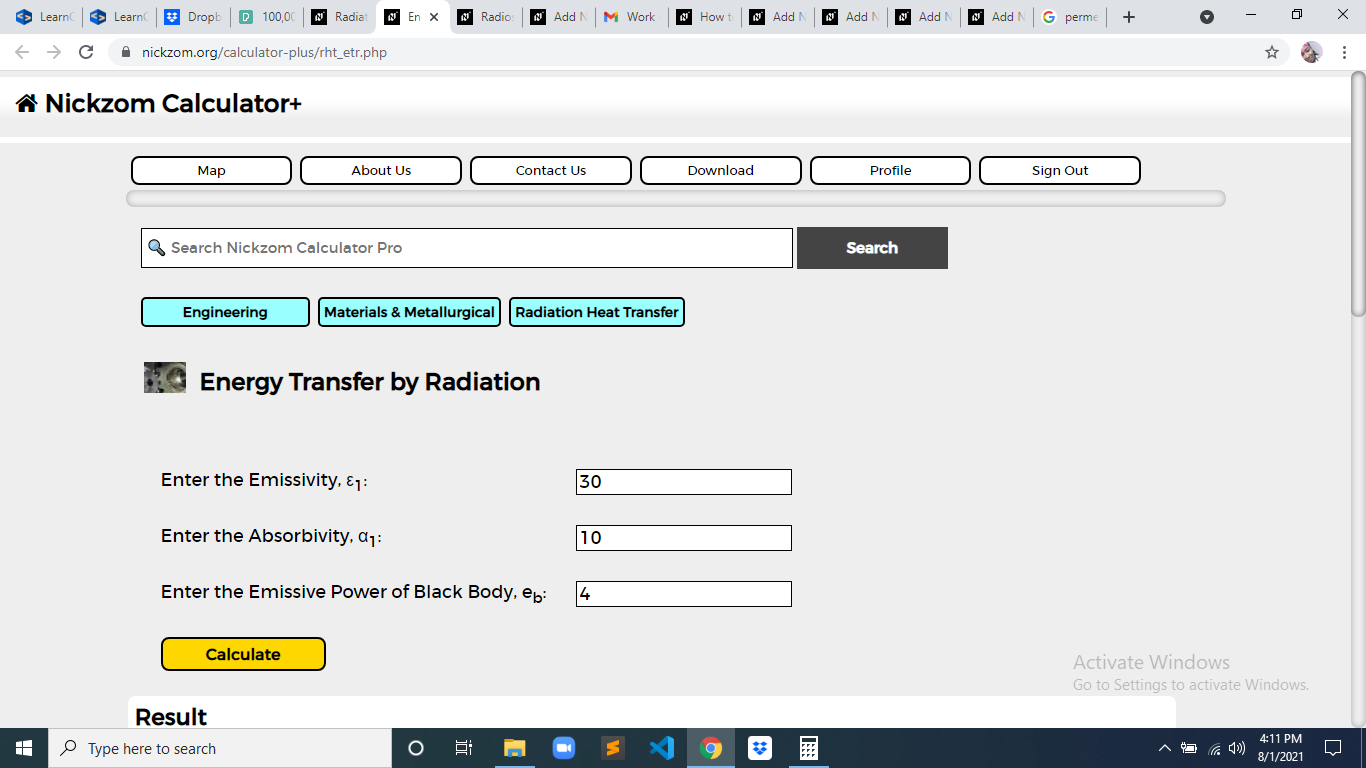Finally, Click on Calculate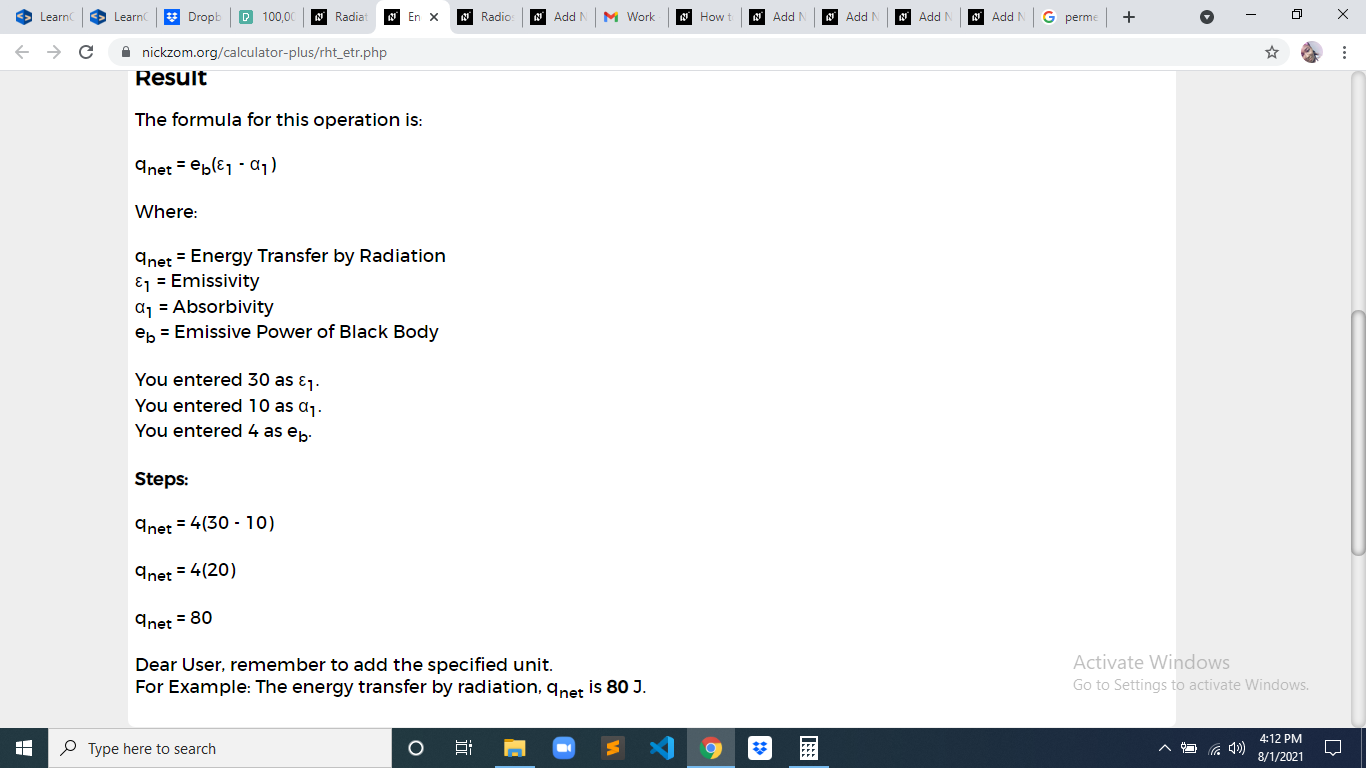As you can see from the screenshot above, Nickzom Calculator– The Calculator Encyclopedia solves for the energy transfer by radiation and presents the formula, workings and steps too.

## How to Calculate and Solve for Radiosity | Radiation Heat Transfer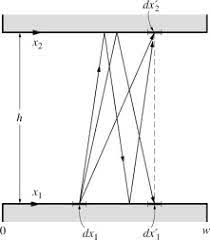To compute for radiosity, three essential parameters are needed and these parameters are Total Emissive Power (e), Reflectivity (ρ) and Total Irradiation (G).

J = e + ρG

Where:

e = Total Emissive Power
ρ = Reflectivity

Let’s solve an example;
Find the radiation heat transfer when the total emissive power is 12, the reflectivity is 10 and the total irradiation is 20.

This implies that;

e = Total Emissive Power = 12
ρ = Reflectivity = 10
G = Total Irradiation = 20

J = e + ρG
J = 12 + (10)(20)
J = 12 + 200
J = 212

Therefore, the radiosity is 212 W/m².

Calculating the Total Emissive Power when the Radiosity, the Reflectivity and the Total Irradiation is Given.

e = J – ρG

Where:

e = Total Emissive Power
ρ = Reflectivity

Let’s solve an example;
Find the total emissive power when the radiation heat transfer is 32, the reflectivity is 10 and the total irradiation is 2.

This implies that;

J = Radiation Heat Transfer = 32
ρ = Reflectivity = 10
G = Total Irradiation = 2

e = J – ρG
e = 32 – (10)(2)
e = 32 – 20
e = 12

Therefore, the total emissive power is 12.

## How to Calculate and Solve for Permeation | Mass Transfer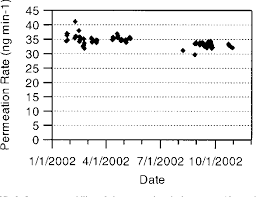The image above represents permeation.

To compute for permeation, six essential parameters are needed and these parameters are Length of Vessel (L), Diameter (D), Initial Concentration (c1), Final Concentration (c2), Inlet Radius of Pipe (r1and Exit Radius of Pipe (r2).

The formula for calculating permeation:

j = -2πLD (c1 – c2 / In(r1/r2))

Where:

j = Permeation
L = Length of Vessel
D = Diameter
c1 = Initial Concentration
c2 = Final Concentration
r1 = Inlet Radius of Pipe
r2 = Exit Radius of Pipe

Let’s solve an example;
Find the permeation when the length of vessel is 8, the diameter is 2, the initial concentration is 4, the final concentration is 9, the inlet radius of pipe is 13 and the exit radius of pipe is 11.

This implies that;

L = Length of Vessel = 8
D = Diameter = 2
c1 = Initial Concentration = 4
c2 = Final Concentration = 9
r1 = Inlet Radius of Pipe = 13
r2 = Exit Radius of Pipe = 11

j = -2πLD (c1 – c2 / In(r1/r2))
j = -2π(8)(2) (4 – 9/In(13/11))
j = (-100.53) (-5/In(1.18))
j = (-100.53) (-5/0.167)
j = (-100.53) (-29.93)
j = 3008.9

Therefore, the permeation is 3008.9 m².

## How to Calculate and Solve for Permeability | Mass Transfer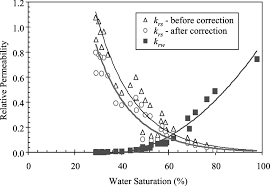The image above represents permeability.

To compute for permeability, five essential parameters are needed and these parameters are Constant (A), Partial Pressure (Po), Activation due to Permeation (Qp), Gas Constant (R) and Temperature (T).

The formula for calculating permeability:

P = APo0.5 . e-Qp/RT

Where:

P = Permeability
A = Constant
Po = Partial Pressure
Qp = Activation due to Permeation
R = Gas Constant
T = Temperature

Let’s solve an example;
Find the permeability when the constant is 12, the partial pressure is 22, the activation due to permeation is 10, the gas constant is 14 and the temperature is 2.

This implies that;

A = Constant = 12
Po = Partial Pressure = 22
Qp = Activation due to Permeation = 10
R = Gas Constant = 14
T = Temperature = 2

P = APo0.5 . e-Qp/RT
P = 12(22)0.5 . e-(10)/(14)(2)
P = 12(4.69) . e-10/28
P = 56.28 . e-0.357
P = 56.28 (0.699)
P = 39.38

Therefore, the permeability is 39.38.

## How to Calculate and Solve for Knudsen Diffusion of Moulding Sand | Mass Transfer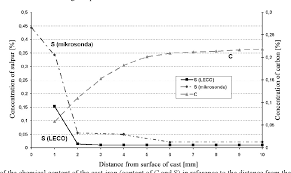The image above represents knudsen diffusion of moulding sand.

To compute for knudsen diffusion of moulding sand, three essential parameters are needed and these parameters are Pore Radius (r), Temperature (T) and Molecular Weight (M).

The formula for calculating knudsen diffusion of moulding sand:

Dk = 9700r√(T/M)

Where:

Dk = Knudsen Diffusion of Moulding Sand
T = Temperature
M = Molecular Weight

Let’s solve an example;
Find the knudsen diffusion of moulding sand when the pore radius is 24, the temperature is 18 and the molecular weight is 12.

This implies that;

r = Pore Radius = 24
T = Temperature = 18
M = Molecular Weight = 12

Dk = 9700r√(T/M)
Dk = 9700(24)√(18/12)
Dk = 9700(24)√(1.5)
Dk = 9700(24)(1.22)
Dk = 285120.6

Therefore, the knudsen diffusion of moulding sand is 285120.6 cm²/s.

Calculating the Pore Radius when the Knudsen Diffusion of Moulding Sand, the Temperature and the Molecular Weight is Given.

r = Dk / 9700 √(T / M)

Where:

Dk = Knudsen Diffusion of Moulding Sand
T = Temperature
M = Molecular Weight

Let’s solve an example;
Find the pore radius when the knudsen diffusion of moulding sand is 20, the temperature is 14 and the molecular weight is 10.

This implies that;

Dk = Knudsen Diffusion of Moulding Sand = 20
T = Temperature = 14
M = Molecular Weight = 10

r = Dk / 9700 √(T / M)
r = 20 / 9700 √(14 / 10)
r = 20 / 9700 √(1.4)
r = 20 / 11446
r = 0.00174

Therefore, the pore radius is 0.00174.